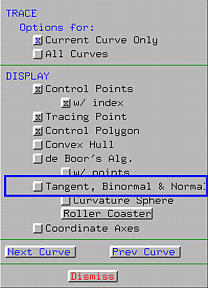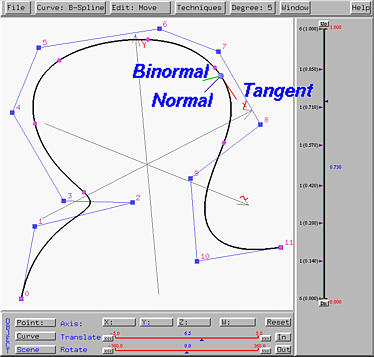The moving triad is a local coordinate system with origin at a point on the curve. The three ``coordinate axes'' are the tangent vector, the binormal vector and the normal vector. The tangent vector gives the direction of movement of the tracing point, the normal vector points the direction the curve is turning, and the binormal vector points to the ``sky'' and is defined to be the vector perpendicular to the tangent and normal with the right-hand system.

This system is capable of displaying the moving triad and as the tracing point moves on the curve the moving triad moves as well. Note that the moving triad should be viewed in space; otherwise, you would only see the tangent and normal vectors because the binormal vector is perpendicular to the screen. Therefore, scene rotation may be required.To display the moving triad, bring up the Display & Tracing Options Window and click on button Tangent, Binormal & Normal. The three vectors will be shown in different colors. In the following figure, the tangent vector is shown in red, the binormal in green and the normal in blue. Note that you can always customize the colors, and as a result, the colors on your system may be different from what are being used on this page. Click here to download a copy of this file space-curve-1.dat for your practice.The following is another triad at a different location. In many cases, it is not entirely clear where these vectors are pointing. Thus, scene rotation may be required to clearly see the directions of these three vectors.